NEET  >  31 Year NEET Previous Year Questions: Chemical Kinetics - 2

# 31 Year NEET Previous Year Questions: Chemical Kinetics - 2

Test Description

## 30 Questions MCQ Test Chemistry Class 12 | 31 Year NEET Previous Year Questions: Chemical Kinetics - 2

31 Year NEET Previous Year Questions: Chemical Kinetics - 2 for NEET 2022 is part of Chemistry Class 12 preparation. The 31 Year NEET Previous Year Questions: Chemical Kinetics - 2 questions and answers have been prepared according to the NEET exam syllabus.The 31 Year NEET Previous Year Questions: Chemical Kinetics - 2 MCQs are made for NEET 2022 Exam. Find important definitions, questions, notes, meanings, examples, exercises, MCQs and online tests for 31 Year NEET Previous Year Questions: Chemical Kinetics - 2 below.
Solutions of 31 Year NEET Previous Year Questions: Chemical Kinetics - 2 questions in English are available as part of our Chemistry Class 12 for NEET & 31 Year NEET Previous Year Questions: Chemical Kinetics - 2 solutions in Hindi for Chemistry Class 12 course. Download more important topics, notes, lectures and mock test series for NEET Exam by signing up for free. Attempt 31 Year NEET Previous Year Questions: Chemical Kinetics - 2 | 30 questions in 60 minutes | Mock test for NEET preparation | Free important questions MCQ to study Chemistry Class 12 for NEET Exam | Download free PDF with solutions
 1 Crore+ students have signed up on EduRev. Have you?
31 Year NEET Previous Year Questions: Chemical Kinetics - 2 - Question 1

### Select the rate law that corresponds to data shown for the following reaction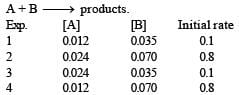Detailed Solution for 31 Year NEET Previous Year Questions: Chemical Kinetics - 2 - Question 1

From data 1 and 3, it is clear that keeping (B) const, When [A] is doubled, rate remains unaffected. Hence rate is independent of [A]. from 1 and 4, keeping [A] constant, when [B] is doubled, rate become 8 times. Hence [rate ∝ [ B]3].

31 Year NEET Previous Year Questions: Chemical Kinetics - 2 - Question 2

### For an exothermic reaction, the energy of activation of the reactants is 

Detailed Solution for 31 Year NEET Previous Year Questions: Chemical Kinetics - 2 - Question 2

Ea(Forward) + ΔH = Ea(back word)
For Exothermic reaction, ΔH = –ve and
∴  activation energy of reactant is less than the energy of activation of products.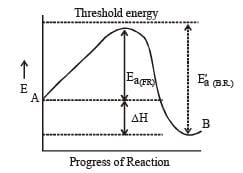31 Year NEET Previous Year Questions: Chemical Kinetics - 2 - Question 3

### A chemical reaction is catalyzed by a catalyst X.Hence X 

Detailed Solution for 31 Year NEET Previous Year Questions: Chemical Kinetics - 2 - Question 3

A catalyst affects equally both forward and backward reactions, therefore it does not affect equilibrium constant of reaction.

31 Year NEET Previous Year Questions: Chemical Kinetics - 2 - Question 4

A substance 'A' decomposes by a first or der reaction starting initially with [A] = 2.00 m and after 200 min, [A] becomes 0.15 m. For this reaction t1/2 is 

Detailed Solution for 31 Year NEET Previous Year Questions: Chemical Kinetics - 2 - Question 4

Given initial concentration (a) = 2.00 m; Time taken (t) = 200 min and final concentration (a – x)    = 0.15 m. For a first order reation rate constant,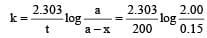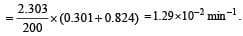Further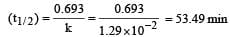31 Year NEET Previous Year Questions: Chemical Kinetics - 2 - Question 5

The rate of reaction depends upon the 

Detailed Solution for 31 Year NEET Previous Year Questions: Chemical Kinetics - 2 - Question 5

The rate of a reaction is the speed at which the reactants are converted into products.
It depends upon the concentration of reactants. e.g for the reaction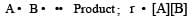31 Year NEET Previous Year Questions: Chemical Kinetics - 2 - Question 6

In a reversible reaction the energy of activation of the forward reaction is 50 kcal. The energy of activation for the reverse reaction will be 

Detailed Solution for 31 Year NEET Previous Year Questions: Chemical Kinetics - 2 - Question 6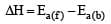Thus energy of activation for reverse reaction depend upon whether reaction is exothermic or endothermic If reaction is exothermic,  ΔH = +ve Ea(b) > Ea(f) If reaction is endothermic  ΔH =+ve Ea(b) < Ea(f )

31 Year NEET Previous Year Questions: Chemical Kinetics - 2 - Question 7

In a reaction, → Product, rate is doubled when the concentration of is doubled, and rate increases by a factor of 8 when the concentrations of both the reactants (and B) are doubled, rate law for the reaction can be written as

Detailed Solution for 31 Year NEET Previous Year Questions: Chemical Kinetics - 2 - Question 7

\\

31 Year NEET Previous Year Questions: Chemical Kinetics - 2 - Question 8

Activation energy of a chemical reaction can be determined by 

Detailed Solution for 31 Year NEET Previous Year Questions: Chemical Kinetics - 2 - Question 8

We know that the activation energy of chemical reaction is given by formula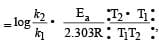where k1 is the rate constant at temperature T1 and k2 is the rate constant at temperature T2 and Ea is the activation energy.  Therefore activation energy of chemical reaction is determined by evaluating rate constant at two different temperatures.

31 Year NEET Previous Year Questions: Chemical Kinetics - 2 - Question 9

Half life of a first order reaction is 4 s and the initial concentration of the reactants is 0.12 M.The concentration of the reactant left after 16 s is 

Detailed Solution for 31 Year NEET Previous Year Questions: Chemical Kinetics - 2 - Question 9

t1/2 = 4 s T = 16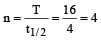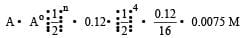where Å = initial concentration &  A = concentration left after time t

31 Year NEET Previous Year Questions: Chemical Kinetics - 2 - Question 10

In the following reaction , how is the rate of appearance of the underlined product related to the rate of disappearance of the underlined reactant ?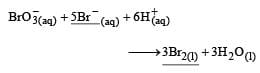Detailed Solution for 31 Year NEET Previous Year Questions: Chemical Kinetics - 2 - Question 10

Rate of reaction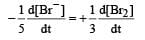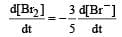31 Year NEET Previous Year Questions: Chemical Kinetics - 2 - Question 11

When a biochemical reaction is carried out in laboratory in the absence of enzyme then rate of reaction obtained is 10–6 times, then activation energy of reaction in the presence of enzyme is

Detailed Solution for 31 Year NEET Previous Year Questions: Chemical Kinetics - 2 - Question 11

The presence of enzyme (catalyst) increases the speed of reaction by lowering the energy barrier, i.e. a new path is followed with lower activation energy.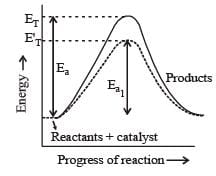Here ET is the threshold energy.
Ea and Ea1 is energy of activation of reaction in absence and presence of catalyst respectively.

31 Year NEET Previous Year Questions: Chemical Kinetics - 2 - Question 12

For the reaction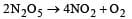rate and rate constant are 1.02 × 10–4 mol lit–1 sec–1 and 3.4 × 10–5 sec–1 respectively then concentration of N2O5 at that time will be 

Detailed Solution for 31 Year NEET Previous Year Questions: Chemical Kinetics - 2 - Question 12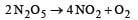from the unit of rate constant it is clear that the reaction follow first order kinetics. Hence by rate law equation,
r = k [N2O5] where r = 1.02 × 10–4,
k = 3.4 × 10–5 1.02 × 10–4 = 3.4 × 10–5 [N2O5]
[N2O5] = 3M

31 Year NEET Previous Year Questions: Chemical Kinetics - 2 - Question 13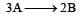, rate of reactionis equal to

Detailed Solution for 31 Year NEET Previous Year Questions: Chemical Kinetics - 2 - Question 13Rate of appearance of B is equal to rate of disappearance of A.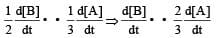31 Year NEET Previous Year Questions: Chemical Kinetics - 2 - Question 14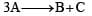, it would be a zero order reaction when

Detailed Solution for 31 Year NEET Previous Year Questions: Chemical Kinetics - 2 - Question 14

For reactionIf it is zero order reaction r = k [A]0, i.e the rate remains same at any concentration of  'A'. i.e independent upon concentration of A.

31 Year NEET Previous Year Questions: Chemical Kinetics - 2 - Question 15

The temperature dependence of rate constant (k) of a chemical reaction is written in terms of Arrhenius equation,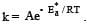. Activation energy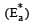of the reaction can be calculated by plotting 

Detailed Solution for 31 Year NEET Previous Year Questions: Chemical Kinetics - 2 - Question 15

k = Ae-Ea / Rt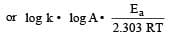Comparing the above equation with y = mx + c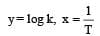Thus A plot of log10k vs 1/T should be a straight line, with slope equal to – Ea/2.303 RT and intercept equal to log A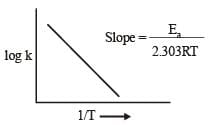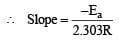or  Ea = –2.303R x Slope

31 Year NEET Previous Year Questions: Chemical Kinetics - 2 - Question 16

If the rate of the reaction is equal to the rate constant, the order of the reaction is 

Detailed Solution for 31 Year NEET Previous Year Questions: Chemical Kinetics - 2 - Question 16

∵ r = k[A]n
if n = 0
r = k[A]0 or  r = k thus for zero order reactions rate is equal to the rate constant.

31 Year NEET Previous Year Questions: Chemical Kinetics - 2 - Question 17

The activation energy for a simple chemical reaction A → B is Ea in forward direction. The activation energy for reverse reaction 

Detailed Solution for 31 Year NEET Previous Year Questions: Chemical Kinetics - 2 - Question 17

The activation energy of reverse reaction will depend upon whether the forward reaction is exothermic or endothermic.
As ΔH = Ea (forward reaction) – Ea(backward reaction)
For exothermic reaction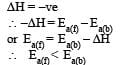for endothermic reaction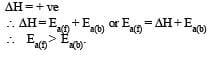31 Year NEET Previous Year Questions: Chemical Kinetics - 2 - Question 18

The reaction A → B follows first order kinetics.The time taken for 0.8 mole of A to produce 0.6 mole of B is 1 hour. What is the time taken for conversion of 0.9 mole of A to produce 0.675 mole of B?

Detailed Solution for 31 Year NEET Previous Year Questions: Chemical Kinetics - 2 - Question 18

A → B For a first order reaction given a = 0.8 mol,    (a – x) = 0.8 – 0.6 = 0.2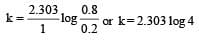again a = 0.9,   a – x = 0.9 – 0.675 = 0.225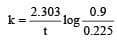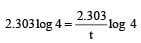Hence t = 1 hour

31 Year NEET Previous Year Questions: Chemical Kinetics - 2 - Question 19

The rate of a first order reaction is 1.5 × 10–2 mol L–1 min–1 at 0.5 M concentration of the reactant.The half life of the reaction is 

Detailed Solution for 31 Year NEET Previous Year Questions: Chemical Kinetics - 2 - Question 19

For a first order reaction, A → products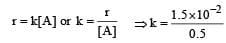= 3 × 10–2

Further,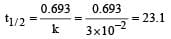31 Year NEET Previous Year Questions: Chemical Kinetics - 2 - Question 20

For a first order reaction A → B th e reaction rate at reactant concentration of 0.01 M is found to be 2.0 x 10-5 mol L-1 S-1. The half life period of the reaction is [2 00 5]

Detailed Solution for 31 Year NEET Previous Year Questions: Chemical Kinetics - 2 - Question 20

Given [A] = 0.01 M
Rate = 2.0 × 10–5 mol L–1 S–1
For a first order reaction Rate = k[A]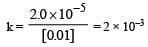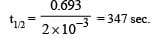31 Year NEET Previous Year Questions: Chemical Kinetics - 2 - Question 21

The rate of reaction between two reactants A and B decreases by a factor of 4 if the concentration of reactant B is doubled. The order of this reaction with respect to reactant B is:

Detailed Solution for 31 Year NEET Previous Year Questions: Chemical Kinetics - 2 - Question 21

RateI  = k [A]x[B]y ... (1)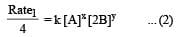or Rate1 = 4k[A]x[2B]y

From (1) and (2) we get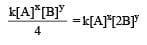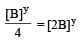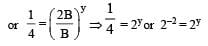or    y = –2.

31 Year NEET Previous Year Questions: Chemical Kinetics - 2 - Question 22

For the reaction 2A + B → 3C + D which of the following does not express the reaction rate ? 

Detailed Solution for 31 Year NEET Previous Year Questions: Chemical Kinetics - 2 - Question 22

In the given options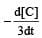will not represent the reaction rate. It should not have –ve sign as it is product. since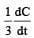show the rate of formation ofproduct C which will be positive.

31 Year NEET Previous Year Questions: Chemical Kinetics - 2 - Question 23

Consider the reaction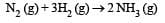The equality relationship between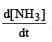and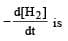Detailed Solution for 31 Year NEET Previous Year Questions: Chemical Kinetics - 2 - Question 23

If we write rate of reaction in terms of concentration of NH3 and H2,then Rate of reaction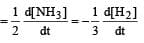So,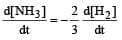31 Year NEET Previous Year Questions: Chemical Kinetics - 2 - Question 24

The reaction of hydrogenandiodine monochloride is given as: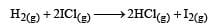The reaction is of first order with respect to H2(g) and ICI(g), following mechanisms were proposed.
Mechanism A: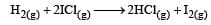Mechanism B: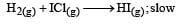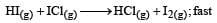Which of the above mechanism(s) can be consistent with the given information about the reaction?

Detailed Solution for 31 Year NEET Previous Year Questions: Chemical Kinetics - 2 - Question 24

As the slowest step is the rate determining step thus the mechanism B will be more consistent with the given information also because it involve one molecule of H2 and one molecule of  ICl it can expressed as r = k [H2][ICl] Which shows that the reaction is first order w.r.t. both H2 & ICl.

31 Year NEET Previous Year Questions: Chemical Kinetics - 2 - Question 25

In a first-order reaction A → B, if k is rate constant and inital concentration of the reactant A is 0.5 M, then the half-life is 

Detailed Solution for 31 Year NEET Previous Year Questions: Chemical Kinetics - 2 - Question 25

For a first order reaction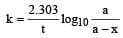when t = t½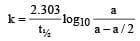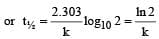31 Year NEET Previous Year Questions: Chemical Kinetics - 2 - Question 26

If 60% of a first order reaction was completed in 60 minutes, 50% of the same reaction would be completed in aproximately 

Detailed Solution for 31 Year NEET Previous Year Questions: Chemical Kinetics - 2 - Question 26

For a first order reaction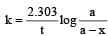when t = 60 and x = 60%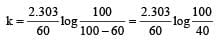Now,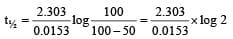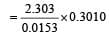=  45.31 min.

31 Year NEET Previous Year Questions: Chemical Kinetics - 2 - Question 27

The rate constants k1 and k2 for two different reactions are 1016 . e–2000/T and 1015 . e–1000/T, respectively. The temperature at which k1 = k2 is :

Detailed Solution for 31 Year NEET Previous Year Questions: Chemical Kinetics - 2 - Question 27

Given,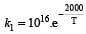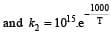when k1 and k2 are equal at any temperature T, we have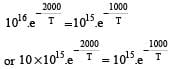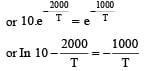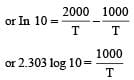or 2.303 ×1×T=1000           [∴ log 10= 1]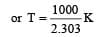31 Year NEET Previous Year Questions: Chemical Kinetics - 2 - Question 28

The bromination of acetone that occurs in acid solution is represented by this equation.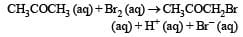These kinetic data were obtained for given reaction concentrations.

Initial Concentrations, M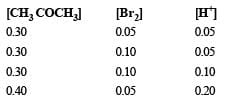Initial rate, disappearance of Br2, Ms–1

5.7×10–5
5.7 × 10–5
1.2 × 10–4
3.1 × 10–4

Base on these data, the rate equations is:

Detailed Solution for 31 Year NEET Previous Year Questions: Chemical Kinetics - 2 - Question 28

Rewriting the given data for the reaction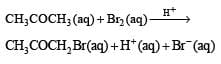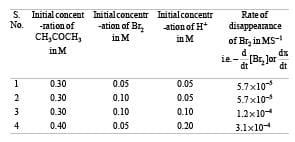Actually this reaction is autocatalyzed and involves complex calculation for concentration terms.
We can look at the above results in a simple way to find the dependence of reaction rate (i.e. rate of disappearance of Br2).
From data (1) and (2) in which concentration of CH3COCH3 and H+ remain unchanged and only the concentration of Br2 is doubled, there is no change in rate of reaction. It means the rate of reaction is independent of concentration of Br2.
Again from (2) and (3) in which (CH3CO CH3) and (Br2) remain constant but H+ increases from 0.05 M to 0.10 i.e. doubled, the rate of reaction changes from 5.7×10–5 to 1.2 × 10–4 (or 12 × 10–5), thus it also becomes almost doubled. It shows that rate of reaction is directly proportional to [H+].
From (3) and (4), the rate should have doubled due to increase in conc of [H+] from 0.10 M to 0.20 M but the rate has changed from 1.2× 10–4 to 3.1×10–4. This is due to change in concentration of CH3 CO CH3 from 0.30 M to 0.40 M. Thus the rate is directly proportional to [CH3 COCH3].
We now get rate = k [CH3COCH3]1[Br2]0[H+]1
= k [CH3COCH3][H+].

31 Year NEET Previous Year Questions: Chemical Kinetics - 2 - Question 29

For the reaction,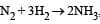,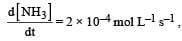the value of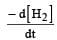would be dt:

Detailed Solution for 31 Year NEET Previous Year Questions: Chemical Kinetics - 2 - Question 29

Rate of disappearance of H2 = rate of formation of NH3.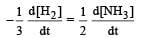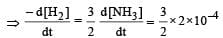= 3 ×10 –4 mol L–1s –1

31 Year NEET Previous Year Questions: Chemical Kinetics - 2 - Question 30

In the reaction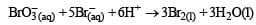The rate of appearance of bromine (Br2) is  related to rate of disappearance of bromide ions as following:

Detailed Solution for 31 Year NEET Previous Year Questions: Chemical Kinetics - 2 - Question 30

Rate of disappearance of Br
= rate of appearance of Br2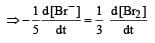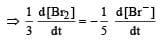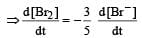## Chemistry Class 12

157 videos|385 docs|261 tests
 Use Code STAYHOME200 and get INR 200 additional OFF Use Coupon Code
Information about 31 Year NEET Previous Year Questions: Chemical Kinetics - 2 Page
In this test you can find the Exam questions for 31 Year NEET Previous Year Questions: Chemical Kinetics - 2 solved & explained in the simplest way possible. Besides giving Questions and answers for 31 Year NEET Previous Year Questions: Chemical Kinetics - 2, EduRev gives you an ample number of Online tests for practice

## Chemistry Class 12

157 videos|385 docs|261 tests

### How to Prepare for NEET

Read our guide to prepare for NEET which is created by Toppers & the best Teachers